# Title 40

## SECTION 1066.270

(a) Overview. Use force measurements to verify the dynamometer's settings based on coastdown procedures.

(b) Scope and frequency. Perform this verification upon initial installation, within 7 days of testing, and after major maintenance.

(c) Procedure. This procedure verifies the dynamometer's settings derived from coastdown testing. For dynamometers that have an automated process for this procedure, perform this evaluation by setting the initial speed, final speed, inertial coefficients, and road-load coefficients as required for each test, using good engineering judgment to ensure that these values properly represent in-use operation. Use the following procedure if your dynamometer does not perform this verification with an automated process:

(1) Warm up the dynamometer as specified by the dynamometer manufacturer.

(2) With the dynamometer in coastdown mode, set the dynamometer inertia for the smallest vehicle weight that you expect to test and set A, B, and C road-load coefficients to values typical of those used during testing. Program the dynamometer to coast down over the dynamometer operational speed range (typically from a speed of 80 mi/hr through a minimum speed at or below 10 mi/hr). Perform at least one coastdown run over this speed range, collecting data over each 10 mi/hr interval.

(3) Repeat the steps in paragraph (c)(2) of this section with the dynamometer inertia and road-load coefficients set for the largest vehicle weight that you expect to test.

(4) Determine the average coastdown force, F, for each speed and inertia setting for each of the coastdowns performed using the following equation: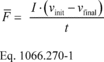Where: F = the mean force measured during the coastdown for each speed interval and inertia setting, expressed in lbf·s 2/ft and rounded to four significant figures. I = the dynamometer's inertia setting, in lbf·s 2/ft. vinit = the speed at the start of the coastdown interval, expressed in ft/s to at least four significant figures. vfinal = the speed at the end of the coastdown interval, expressed in ft/s to at least four significant figures. t = coastdown time for each speed interval and inertia setting, accurate to at least 0.01 s. Example: I = 2000 lbm = 62.16 lbf·s 2/ft vinit = 25 mi/hr = 36.66 ft/s vfinal = 15 mi/hr = 22.0 ft/s t = 5.00 s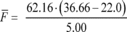F = 182.2 lbf

(5) Calculate the target value of coastdown force, Fref, based on the applicable dynamometer parameters for each speed interval and inertia setting.

(6) Compare the mean value of the coastdown force measured for each speed interval and inertia setting, Fact, to the corresponding Fref to determine values for coastdown force error, Ferror, using the following equation: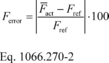Example: Fref = 192 lbf Fact = 191 lbf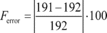Ferror = 0.5%

(d) Performance evaluation. The coastdown force error determined in paragraph (c) of this section may not exceed the following:

(1) For vehicles at or below 20,000 pounds GVWR, the maximum allowable error, Ferrormax, for all speed intervals and inertia settings is 1.0% or the value determined from Eq. 1066.270-3, whichever is greater.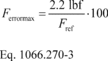Example: Fref = 192 lbf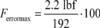Ferrormax = 1.14%

(2) For vehicles above 20,000 pounds GVWR, the maximum allowable error, Ferrormax, for all speed intervals and inertia settings is ±1.0% or ±39.2 N, whichever is greater.

(e) Remedy for nonconforming dynamometers. If the dynamometer is not able to meet this requirement, diagnose and repair the dynamometer before continuing with emission testing. Diagnosis should include performing the verifications in § 1066.255 and § 1066.260.

[79 FR 23823, Apr. 28, 2014, as amended at 80 FR 9120, Feb. 19, 2015; 81 FR 74201, Oct. 25, 2016]